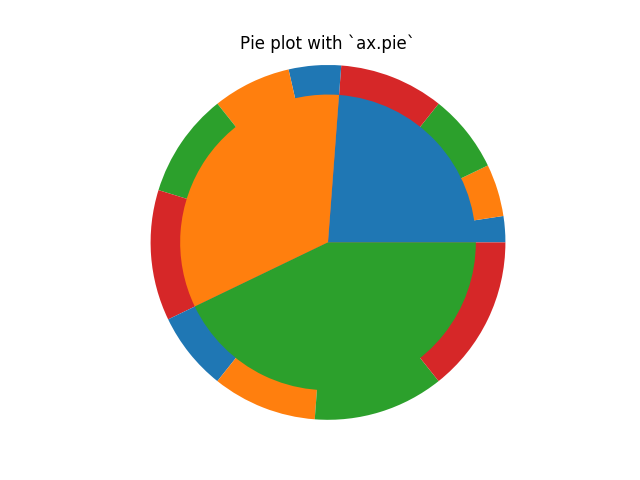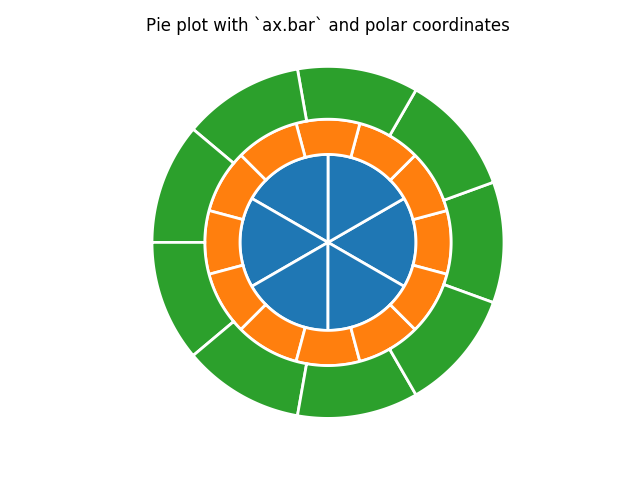Travis-CI:# Nested pie charts¶

The following examples show two ways to build a nested pie chart in Matplotlib.

```from matplotlib import pyplot as plt
import numpy as np
```

The most straightforward way to build a pie chart is to use the `pie method`

In this case, pie takes values corresponding to counts in a group. We’ll first generate some fake data, corresponding to three groups. In the outer circle, we’ll treat each number as belonging to its own group. In the inner circle, we’ll plot them as members of their original 3 groups.

```vals = np.array([[1, 2, 3, 4], [2, 3, 4, 5], [3, 4, 5, 6]])
fig, ax = plt.subplots()
colors=plt.rcParams["axes.prop_cycle"].by_key()["color"][:vals.shape])
ax.set(aspect="equal", title='Pie plot with `ax.pie`')

plt.show()
```However, you can accomplish the same output by using a bar plot on axes with a polar coordinate system. This may give more flexibility on the exact design of the plot.

In this case, we need to map x-values of the bar chart onto radians of a circle.

```fig, ax = plt.subplots(subplot_kw=dict(polar=True))

left_inner = np.arange(0.0, 2 * np.pi, 2 * np.pi / 6)
left_middle = np.arange(0.0, 2 * np.pi, 2 * np.pi / 12)
left_outer = np.arange(0.0, 2 * np.pi, 2 * np.pi / 9)

ax.bar(x=left_inner,
width=2 * np.pi / 6, bottom=0, color='C0',
linewidth=2, edgecolor='w',
height=np.zeros_like(left_inner) + 5)

ax.bar(x=left_middle,
width=2 * np.pi / 12, bottom=5, color='C1',
linewidth=2, edgecolor='w',
height=np.zeros_like(left_middle) + 2)

ax.bar(x=left_outer,
width=2 * np.pi / 9, bottom=7, color='C2',
linewidth=2, edgecolor='w',
height=np.zeros_like(left_outer) + 3)

ax.set(title="Pie plot with `ax.bar` and polar coordinates")
ax.set_axis_off()
plt.show()
```Total running time of the script: ( 0 minutes 0.049 seconds)

Gallery generated by Sphinx-Gallery